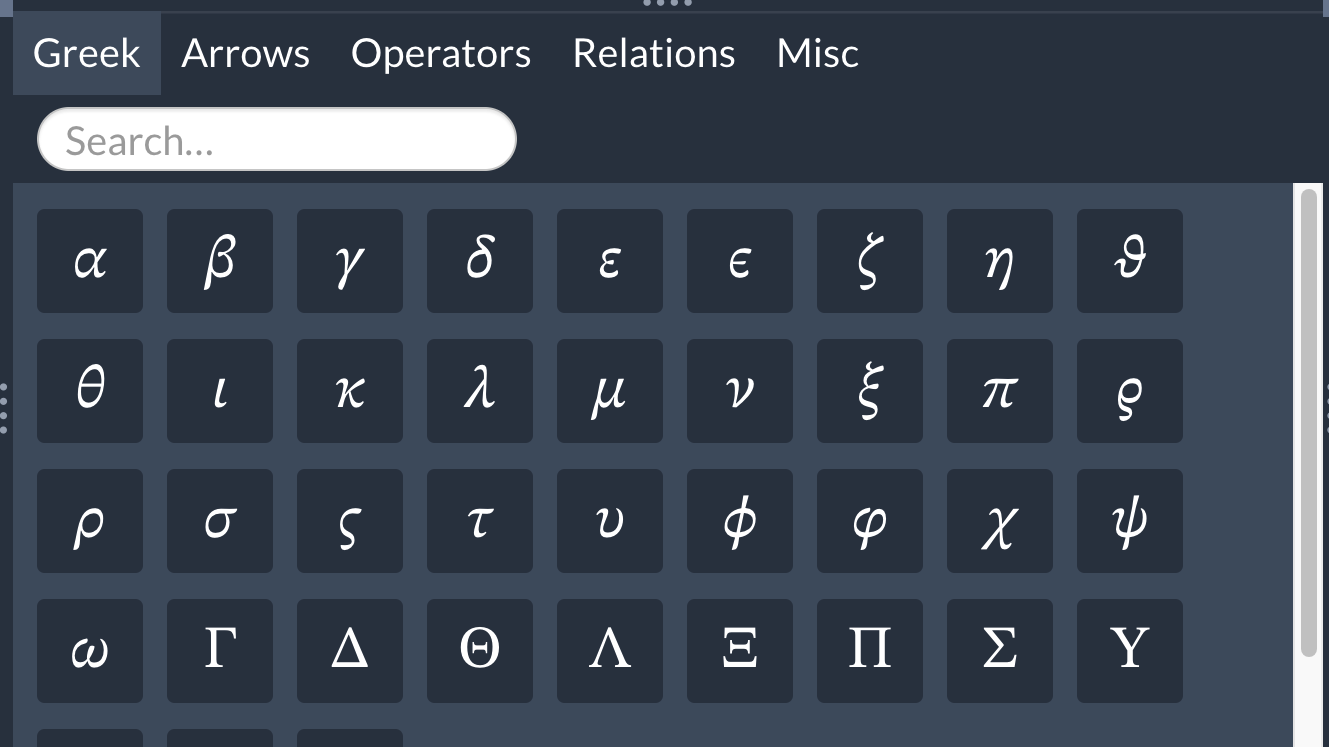Did you know? Overleaf has a symbol palette for users with premium accounts. Read more here. Note that some of the symbols require loading of the amssymb package, and this information is shown when you hover on the symbol.## Greek letters

 $\alpha A$\alpha A $\nu N$\nu N $\beta B$\beta B $\xi \Xi$\xi \Xi $\gamma \Gamma$\gamma \Gamma $oO\;$o O $\delta \Delta$\delta \Delta $\pi \Pi$\pi \Pi $\epsilon \varepsilon E\;$\epsilon \varepsilon E $\rho \varrho P\;$\rho \varrho P $\zeta Z$\zeta Z $\sigma \,\!\Sigma \;$\sigma \Sigma $\eta H$\eta H $\tau T$\tau T $\theta \vartheta \Theta$\theta \vartheta \Theta $\upsilon \Upsilon$\upsilon \Upsilon $\iota I$\iota I $\phi \varphi \Phi$\phi \varphi \Phi $\kappa K$\kappa K $\chi X$\chi X $\lambda \Lambda \;$\lambda \Lambda $\psi \Psi$\psi \Psi $\mu M$\mu M $\omega \Omega$\omega \Omega

## Arrows

 $\leftarrow$\leftarrow $\Leftarrow$\Leftarrow $\rightarrow$\rightarrow $\Rightarrow \;$\Rightarrow $\leftrightarrow$\leftrightarrow $\rightleftharpoons$\rightleftharpoons $\uparrow$\uparrow $\downarrow$\downarrow $\Uparrow \;$\Uparrow $\Downarrow$\Downarrow $\Leftrightarrow \;$\Leftrightarrow  $\Updownarrow$\Updownarrow $\mapsto$\mapsto $\longmapsto \;$\longmapsto $\nearrow$\nearrow $\searrow$\searrow $\swarrow$\swarrow  $\nwarrow$\nwarrow $\leftharpoonup$\leftharpoonup  $\rightharpoonup$\rightharpoonup $\leftharpoondown$\leftharpoondown  $\rightharpoondown$\rightharpoondown

## Miscellaneous symbols

 $\infty \;\;$\infty $\forall \;$\forall $\Re$\Re $\Im$\Im $\nabla$\nabla $\exists$\exists $\partial$\partial $\nexists$\nexists $\emptyset$\emptyset $\varnothing \;$\varnothing $\wp$\wp $\complement$\complement $\neg$\neg $\cdots$\cdots $\square$\square $\surd$\surd  $\blacksquare$\blacksquare $\triangle$\triangle

## Binary Operation/Relation Symbols

 $\times$\times $\cdot$\cdot $\div$\div $\cap$\cap $\cup$\cup $\neq \;$\neq $\leq$\leq $\geq$\geq  $\in$\in $\perp \;$\perp $\notin$\notin $\subset$\subset $\simeq$\simeq $\approx$\approx $\wedge$\wedge $\vee$\vee $\oplus \;$\oplus $\otimes$\otimes $\Box$\Box $\boxtimes$\boxtimes $\equiv$\equiv $\cong$\cong